# Casson MHD Nano Fluid Flow with Internal Heat Generation and Viscous Dissipation of an Exponential Stretching Sheet

Casson MHD Nano Fluid Flow with Internal Heat Generation and Viscous Dissipation of an Exponential Stretching Sheet

M.C. Kemparaju B. LavanyaMahantesh M. Nandeppanavar N. Raveendra

Department of Mathematics, Jyothy Institute of Technology (CIIRC), Bangalore 560062, India

Department of Mathematics, Manipal Institute of Technology, Manipal Academy of Higher Education, Manipal, Karnataka 576104, India

Department of Mathematics, Government College (Autonomous), Kalaburagi 585104, India

Department of Mathematics, Rajarajeswari College of Engineering, Bangalore 560074, India

Corresponding Author Email:
lavanya.b@manipal.edu
Page:
767-772
|
DOI:
https://doi.org/10.18280/ijht.400315
13 May 2022
|
Accepted:
12 June 2022
|
Published:
30 June 2022
| Citation

OPEN ACCESS

Abstract:

The present paper examines Casson MHD nanofluid flow with internal heat generation and viscous dissipation of an exponentially stretching sheet. The conditions depicting the hydromagnetic flow, heat and mass transfer of a thick nanofluid over an isothermal extending sheet are tackled numerically by applying 4th order RK shooting strategy. The governing PDE’s of flow, heat and concentration profiles are transformed into an arrangement of nonlinear ODE’s by using similarity transformations. The impact of heat transfer, Prandtl number, thermpphorosis, Brownian motion and Lewis number on flow, energy, concentration profiles and physical quantities are analyzed and displayed through graphs and tables. It is observed that, the flow rate decrease with increase in magnetuc field and enhance in Eckert number both energy and mass transfer rate increases.

Keywords:

Casson, nano fluid, viscous dissipation, exponential, heat generation

1. Introduction

A nanofluid is a fluid containing nanometer-sized particles, called nanoparticles. These fluids are engineered colloidal suspensions of nanoparticles in a base fluid. The nanoparticles used in nanofluids are typically made of metals, oxides, carbides, or carbon nanotubes. Common base fluids include water, ethylene glycoland oil. Nanofluids have novel properties that make them potentially useful in many applications in heat transfer including microelectronics, fuel cells, pharmaceutical processes, and hybrid-powered engines, engine cooling/vehicle thermal management, domestic refrigerator, chiller, heat exchanger, in grinding, machining and in boiler flue gas temperature reduction. Okonkwo et al.  concentrated in the paper A refreshed survey of nanofluids in different warmth move gadgets. The suspension of these strong particles in the base liquid upgrades the energy transmission in the liquid prompting improved warm conductivity properties and better warmth move qualities The resultant liquids have been believed to have higher upsides of warm conductivity studied by Ganji et al. . Okonkwo et al.  studied Comparison of experimental and theoretical methods of obtaining the thermal properties of alumina/iron mono and hybrid nanofluids. Warmth and mass exchange examination of nanofluid stream dependent on and over a moving pivoting plate and effect of different nanoparticle shapes concentrated by Abbas and Magdy .

Warmth age, circulate and equilibrium are significant issues in both low and excessive temperature power gadgets. A low temperature PEMFC could not withstand an excessive temperature because the polymer electrolyte depends on fluid water to hold it dynamic. Impacts of warm radiation, heat age and actuated appealing discipline on hydromagnetic loose convection move of couple stress liquid in an isoflux-isothermal vertical channel focused by using Zaidi et al. . Khan et al.  has examined Importance of heat age in synthetically responsive stream exposed to convectively warmed floor. Dissolving MHD stagnation point flow and heat flow of a nano liquid with Non-directly heat radiation and substance reaction concentrated by Kemparaju et al. . Lavanya  tested Hall current and heat radiation influences on warmth and mass exchange of temperamental MHD movement of a viscoelastic micropolar liquid via a permeable medium. Noghrehabadi et al.  taken into consideration warmth flow of magnetohydrodynamic viscid nanofluids over an isothermal growing sheet. Shaky MHD three-dimensional Casson nanofluid move over a permeable straight extending sheet with slip situation. Wildernesses in warm temperature and mass trade concentrated by way of Mondal et al. . Acharya et al.  tested Influence of slanted appealing discipline on the progression of consolidated nanomaterial over a risky surface: the mixture notion. This sort of three papers likewise utilized for contrasting our effects.

As of late, there has been an expanding interest in the blood stream of non-Newtonian liquids having a yield pressure. Casson liquid is the main non-Newtonian liquid which has limited yield pressure. In view of this Sarifuddin  study the reaction of mass exchange of blood moving through sporadic and cosine-molded stenoses in the shaky state, i.e., CFD. Displaying of Casson Liquid Stream and Mass Vehicle Through Atherosclerotic Vessels. Sohail et al.  considered Entropy age in MHD Casson liquid stream with variable warmth conductance and warm conductivity over non-straight bi-directional extending surface. in this paper creator features the assets of force, entropy age, species and warm spread on limit layer stream (BLF) of Casson fluid over a straightly lengthening surface considering radiation and Joule warming impacts critical. Transportation of warm and species are offered by utilizing the temperature-subordinate models of warm conductivity and mass dispersion coefficient. On transport of receptive solute in a pulsatile Casson liquid move through an annulus This paper inspects the impact of heterogeneous compound response on the vehicle of a solute in a Casson liquid course through an annular line under an intermittent pressing factor inclination concentrated by Debnath et al. . Raju and KVS  considered MHD Casson liquid course through an upward plate in this examination, impacts of various actual amounts like dispersal, warm radiation, and prompted attractive field on magnetohydrodynamic Casson liquid move through an upward plate is tended to. Radiation Impact on MHD Casson Liquid Stream Directly Permeable Extending Sheet in the Presence Compound Response concentrated by Swapna et al. . Gharami et al.  studied analytical and numerical solution of viscous fluid flow with the effects of thermal radiation and chemical reaction past a vertical porous surface.

Apparently, concentrates on a Casson MHD Nano liquid circulation with a dramatically rotating internal warm temperature age and gooey dissemination i.e., presence of thick dispersal has now not been idea about up until this point. Nonetheless, in those examinations, the liquid stream models are characterised as a solitary differential situation with restrictions inside the type of limitations researched. While thinking about a course of motion of coupled nonlinear sport plan of differential situations, for instance, the version provided in this newsletter, the check skilled inside the wake of completing the likeness modifications, is the shooting calculation needed to acquire the essential mathematical preparations without certain underlying conditions. The overseeing conditions modified as normal differential situations making use of fitting likeness adjustments and settled mathematically. The impact of particular dimensionless boundaries on 3 commonplace profiles (pace, temperature and fixation) are talked about and seemed in Tables and diagrams.

2. Mathematcal Formulation

Considered geometry of the present study, in this u and v are velocity components along the rectangular coordinate system x and y. The temperature of the unknown plate wall and the ambient fluid temperature are believed to be Tw & T.

This result in a uniform concentration of ambient fluid C, an unknown plate wall concentration Cw and applied transfer magnetic field B(x) along y-axis are assumed. Except for density, fluid properties are considered to be invariant, changing only in response to changes in the flow. The sheet is assumed to possess a stretch cover Uw=cx. The boundary layer equations that govern it are dimensional in nature.

$~\frac{\partial u}{\partial x}+\frac{\partial u}{\partial y}=0$    (1)

$u\frac{\partial u}{\partial x}+v\frac{\partial u}{\partial y}=\left( 1+\frac{1}{\beta } \right)\upsilon \frac{{{\partial }^{2}}u}{\partial {{y}^{2}}}-\frac{\sigma {{B}^{2}}}{{{\rho }_{f}}}u$     (2)

\begin{align} & u\frac{\partial T}{\partial x}+v\frac{\partial T}{\partial y}=\alpha \frac{{{\partial }^{2}}T}{\partial {{y}^{2}}}+\frac{\text{ }\!\!\mu\!\!\text{ }}{\text{ }\!\!\rho\!\!\text{ }{{\text{C}}_{\text{p}}}}{{\left( \frac{\partial u}{\partial \text{y}} \right)}^{2}} \\ & +\tau \left\{ {{D}_{B}}\frac{\partial c}{\partial y}\frac{\partial T}{\partial y}+\frac{{{D}_{T}}}{{{T}_{\infty }}}{{\left( \frac{\partial T}{\partial y} \right)}^{2}} \right\}+Q \\\end{align}     (3)

$u\frac{\partial C}{\partial y}+v\frac{\partial C}{\partial y}={{D}_{B}}\frac{{{\partial }^{2}}C}{\partial {{y}^{2}}}+\frac{{{D}_{T}}}{{{T}_{\infty }}}\frac{{{\partial }^{2}}T}{\partial {{y}^{2}}}$     (4)

The appropriate boundary conditions are: v=0, u=Uw(x), T=Tw, $D_{B} \frac{\partial c}{\partial y}=-\frac{D_{T}}{T_{\infty}} \frac{\partial T}{\partial y}$  at y=0, u→0, TT, CC as y→0.

The keep going term on the right-hand side of the energy condition is the interior warmth age term. It is characterized with the end goal that it rots dramatically and it is of structure:

$Q=\frac{{{Q}_{0}}({{T}_{w}}-{{T}_{\infty }})}{\rho {{C}_{p}}}\exp \left( -ny\sqrt{\frac{C}{v}} \right)$     (5)

where, n is that the density of the bottom fluid, σ is that the electrical conductivity of the fluid, α is that the thermal diffusivity, Q0 is the space-dependent internal heat generation and n is that the coefficient of absorption. The Eqns. (1)-(4) are expressed in dimensionless form by introducing the following stream function: $u=\frac{\partial \psi}{\partial y}$ and $v=-\frac{\partial \psi}{\partial x}$.

Where as the stream work and subsequently the dimensionless factors are presented inside the accompanying structure:

$\psi ={{\left( C\upsilon \right)}^{1/2}}xF\left( \eta \right)$, $\eta ={{\left( \frac{c}{\upsilon } \right)}^{1/2}}y$,

$\theta (\eta )=\frac{T-{{T}_{\infty }}}{{{T}_{w}}-{{T}_{\infty }}}$, $\phi (\eta )=\frac{C-{{C}_{\infty }}}{{{C}_{w}}-{{C}_{\infty }}}$     (6)

Here, the progression condition is fulfilled preferably. Utilizing the likeness changes, the accompanying arrangement of normal differential conditions is acquired:

$\left( 1+\frac{1}{\beta } \right)F'''+FF''-F{{'}^{2}}-MF'=0$     (7)

${{\theta }^{''}}+Pr\left[ F\theta '+Nb{{\theta }^{'}}{{\phi }^{'}}+Nt\theta {{'}^{2}}+EcF'{{'}^{2}}+\gamma Exp\left( -n\eta \right) \right]=0$     (8)

$\phi ''+LeF\phi '+\frac{Nt}{Nb}\theta ''$     (9)

Subject to the limitations imposed by the boundaries:

$F=0, F=1, \theta=1, N b \varphi^{\prime}=-N t \theta^{\prime}$ at $y=0$

$F=0, \theta=0, \varphi=0$ as $y \rightarrow \infty$     (10)

where, $M=\frac{\sigma B^{2}}{C \rho_{f}}, \operatorname{Pr}=\frac{v}{\alpha}, N b=\tau D_{B} \frac{C_{w}-C_{\infty}}{v}, N t=\tau D_{T} \frac{T_{w}-T_{\infty}}{v T_{\infty}}$, $\gamma=\frac{Q_{0}}{C \rho_{f} C_{p}}, L e=\frac{v}{D_{B}}$ and $E c=\frac{c x^{2}}{T_{w}-T_{\infty}}$ are attractive field boundary, Prandtl number, Brownian movement, thermophoresis, inward warmth source boundaries, and Lewis number, separately.

3. Mathematical Method of Solution

Eq. (7)-(9) have been tackled mathematically with boundary conditions from Eq. (10) along with shooting technique. By utilizing seventh order Runge- Kutta integration method, first we convert the original ODE’s into first order ODE’s by using below Eq. (11):

$\left.\begin{array}{l}p_{1}=F, p_{2}=F^{\prime}, p_{3}=F^{\prime \prime}, p_{4}=\theta, p_{5}=\theta^{\prime}, p_{6}=\phi, p_{7}=\phi^{\prime} \\ p_{1}^{\prime}=p_{2}, \\ p_{2}^{\prime}=p_{3}, \\ p_{3}^{\prime}=\left(1+\frac{1}{\beta}\right)\left(-p_{1} p_{3}+p_{2}^{2}-M p_{2}\right), \\ p_{4^{\prime}}=p_{5}, \\ p_{5}^{\prime}=-\operatorname{Pr}\left(p_{1} p_{5}+E c p_{3}^{2}+N b p_{5} p_{7}+N t p_{5}^{2}+\gamma \exp (-n \eta)\right), \\ p_{6}^{\prime}=p_{7}, \\ p_{7}^{\prime}=-\left(\begin{array}{l}\operatorname{Le} p_{1} p_{7}-\left(\frac{N t}{N b}\right) \\ \operatorname{Pr}\left(p_{1} p_{5}+E c p_{3}^{2}+N b p_{5} p_{7}+N t p_{5}^{2}+\gamma \exp (-n \eta)\right)\end{array}\right)\end{array}\right\}$     (11)

The initial conditions that apply are as follows:

\left. \begin{align} & {{p}_{1}}(0)=0,\,\,\,\,\,\,\,\,\,{{p}_{2}}(0)=1\,,\,\,\,\,\,\,{{p}_{4}}(0)=1,\,\,\,\,\,\,\,\,{{p}_{7}}(0)=-\left( \frac{Nt}{Nb} \right){{p}_{5}}(0) \\ & {{p}_{2}}(\infty )=0,\,\,\,\,\,\,\,{{p}_{4}}(\infty )=0,\,\,\,\,\,{{p}_{6}}(\infty )=0 \\\end{align} \right\}       (12)

After choosing proper guess values for u1=F''(0), u2=θ'(0) and u3=ϕ'(0), the integration is completed. By Newton’s iterative method to compute the values of u1, u2and u3 such that the solutions satisfy the boundary conditions at infinity with minimum error.

4. Results and Discussion

The significance of expanding the inside heat creation in the electromagnetic nanofluid has been considered utilizing the strategy for terminating techniques. In Tables 1 and a couple, correlations of arrangements utilizing the shooting strategy technique, the current writing were in this manner given a neighborhood line see strategy.

Table 1. Comparison of skin friction for different values of M

 M Present results Oyelakin et al.  0 0.99999995 1.00001 0.5 1.22474481 1.22474 1 1.41421349 1.41421 1.5 1.58113875 1.58114

Table 2. Comparison of Nusselt number for distinct estimations of Pr

 Pr Present Results Oyelakin et al.  Acharya et al.  Noghrehabadadi et al.  0.7 0.45391669 0.45391 0.453962 0.453916 2.0 0.91135756 0.91135 0.911397 0.911358 7.0 1.89540334 1.8954 1.895420 1.895404 20.0 3.35390426 3.35390 3.353861 3.353917 70.0 6.46219988 6.462190 --- 6.462290

The arrangements in Table 1 give great consistency of up to 5 decimal spots for precision. In Table 2, the Prandtl mathematical boundary is extraordinary and the ascent inside the boundary expands skin awkwardness, heat, and subsequently weight move. These discoveries are reliable with assumptions, for instance [9, 10, 11] report comparable outcomes. On account of mass exchange, just the circumstance is effectively controlled. The PC status of the mass exchange isn't considered because of the zero nanoparticle transition at the limit.

In Figure 1 for comparison of velocity with η of variable M display values rising from the values of the magnetic parameter decreases at the speed level velocity, it appears that the velocity is moving the sheet decreases with the increase of the unstable parameter M and this means a decrease corresponding to the size of the pressure boundary layer. Figure 2 temperature compared to η the converted values of the displays that rise in magnetic field increases the temperature in all the values of Assumption M, the temperature is found to decrease independently at a distance θ(η) from the sheet. It is noteworthy that the effect of M on temperature profiles is more pronounced than velocity in profiles. Figure 3 compared to η of converted values showing the numerical effects of Prandtl Pr in the temperature profile. Temperature decreases with Pr where there is a constant earth temperature change. An increase in the number of Prandtl reduces the size of the thermal border layer. The Prandtl number indicates the degree of severity of the temperature difference. In the heat transfer components, the Prandtl Pr number controls the equilibrium intensity of the pressure and the thermal boundary layers.

## 1.png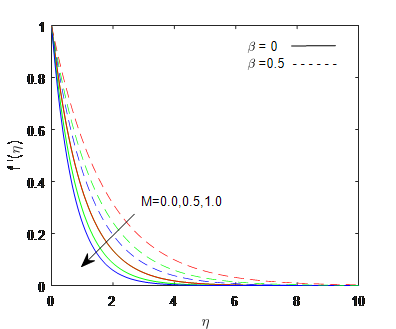Figure 1. f'(η) versus η for changed points of M when Le=1, Pr=1, Ec=0.5, γ=0.5, Nb=Nt=0.2 & n=0.5

## 2.png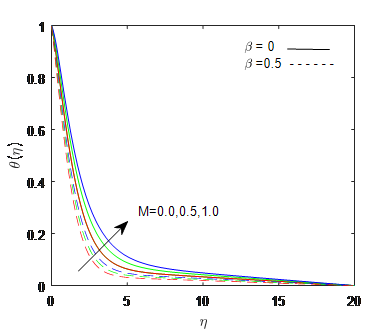Figure 2. θ(η) versus η for changed points of M when Le=1, Pr=1, Ec=0.5, γ=0.5, Nb=Nt=0.2 & n=0.5

When the Prandtl number Pr is small, heat diffuses fast in comparison to velocity (momentum), implying that the thermal boundary layer in liquid metals is significantly thicker than the momentum boundary layer. Heat can diffuse from the sheet quicker in fluids with a lower Prandtl number (and thicker thermal boundary layer structures) than in fluids with a higher Prandtl number. As a result, the Prandtl number may be employed to boost the cooling rate in conducting flows. Figure 4 velocity versus η for changed upsides of pr demonstrates expansion in Prandtl number decreases the focus level. Figure 5 Temperature versus η for changed upsides of γ addresses expansion in inside source boundary diminishes the temperature. Figure 6 indicates Temperature versus η for changed upsides of γ and it shows the decrease in temperature. Figure 7 for changed upsides of ponders the effects of Brownian movement. On nanoparticle focus in the limit layer domain. This figure shows that the Brownian movement decays the both concentration (varieties are little if there should arise an occurrence of bigger values of boundary) also, solutal limit layer thickness. It is due to the way that augmentation in Brownian movement accelerates the arbitrary development which scatter the nanoparticles and hence fixation diminishes.

## 3.png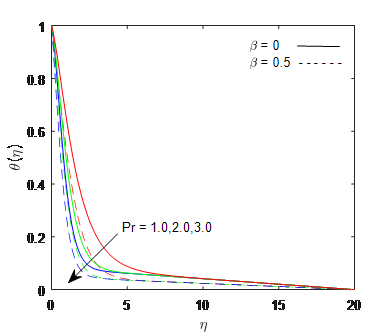Figure 3. θ(η) versus η for changed points of Pr when Le=1, M=0.2, Ec=0.5, γ=0.5, Nb=Nt=0.2 & n=0.5

## 4.png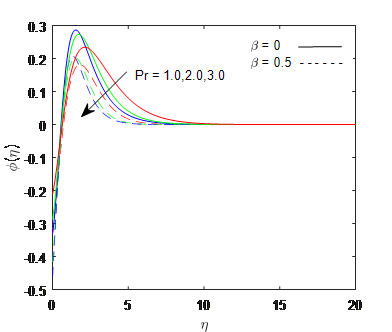Figure 4. ϕ(η) versus η for changed points of Pr when Le=1, M=0.2, Ec=0.5, γ=0.5, Nb=Nt=0.2 & n=0.5

Figure 8 shows the variation of temperature for changed upsides of Nt and Figure 9 represents concentration versus η for changed upsides of Nt The varieties in temperature profile against thermophoresis boundary are explained in Fig. 8 Since thermophoresis wonder speeds up the particles from more blazing locale to cooler locale, thus, heat moves quickly from more blazing surface to liquid, and subsequently, it builds the temperature. The current figure endorsed the above-characterized hypothesis, and furthermore, for given upsides of thermophoresis boundary, a gradually rising temperature profile is shown inside the warm limit layer. The outcomes of thermophoresis on focus profile are appeared in Figure 9. This figure shows that the focus profile increments quickly for bigger upsides of thermophoresis boundary at all marks of stream space.

## 5.pngFigure 5. θ(η) versus η for changed points of γ when Le=1, M=0.2, Ec=0.5, Pr=1, Nb=Nt=0.2 & n=0.5

## 6.png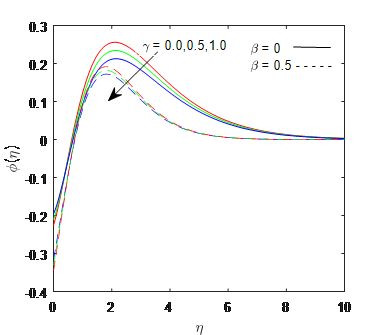Figure 6. ϕ(η) versus η for changed points of γ when Le=1, M=0.2, Ec=0.5, Pr=1, Nb=Nt=0.2 & n=0.5

## 7.png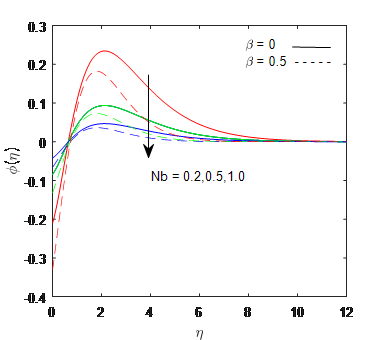Figure 7. ϕ(η) versus η for changed points of Nb when Le=1, M=0.2, Ec=0.5, Pr=1, γ=0.5, Nt=0.2 & n=0.5

Whereas Figure 10 shows temperature versus η for changed values of Ec and Figure 11 depicts concentration versus η for changed values of represents increase in Eckert number increases both temperature and concentration levels it indictes that from the figures that an increase in the Eckert number enhances the flow and thermal boundary layer thickness. This is due to the fact that an increase in dissipation improves the thermal conductivity of the flow. This helps to enhance the thermal and momentum boundary layers Figure 12 θ(η) versus η for changed values of n and Figure 13 concentration versus Lewis number for changed increased values of Le decreases the concentraton level.

## 8.pngFigure 8. θ(η) versus η for changed points of Nt when Le=1, M=0.2, Ec=0.5, Pr=1, γ=0.5, Nb=0.2 & n=0.5

## 9.pngFigure 9. ϕ(η) versus η for changed points of Nt when Le=1, M=0.2, Ec=0.5, Pr=1, γ=0.5, Nb=0.2 & n=0.5

## 10.png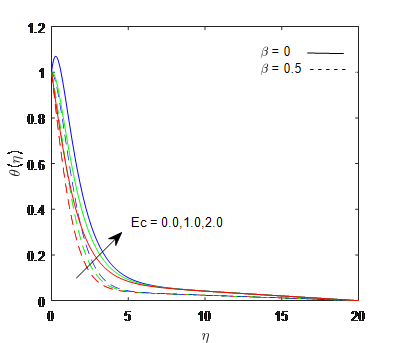Figure 10. θ(η) versus η for changed points of Ec when Le=1, M=0.2, Nt=0.2, Pr=1, γ=0.5, Nb=0.2 & n=0.5

For every boundary, it is seen that the effect is similar to for both limit types, besides near the precarious edge of the divider. Near the very edge of the divider, the focus limit for the condition is consistently diminishing and moves toward zero at vastness. it had been likewise noticed that the nanoparticle profiles initially decrease at that point increment in light of the fact that the attractive motion, Prandtl number, nanoparticle properties and inside warmth source limits increase. This might be credited to the free nanoparticle advancement and in like manner the thermophoresis scattering. This insight stays veritable PC conditions. A requirement of using the PC condition is that the strange starting negative obsession near the divider. Far away from the divider, the results are at any rate as demonstrated by existing composition.

## 11.png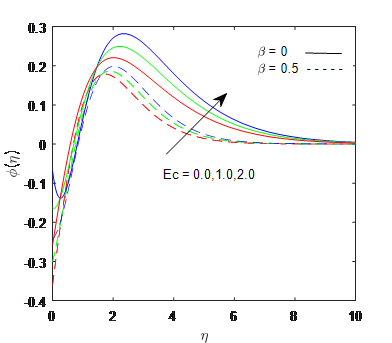Figure 11. ϕ(η) versus η for changed points of Ec when Le=1, M=0.2, Nt=0.2, Pr=1, γ=0.5, Nb=0.2 & n=0.5

## 12.png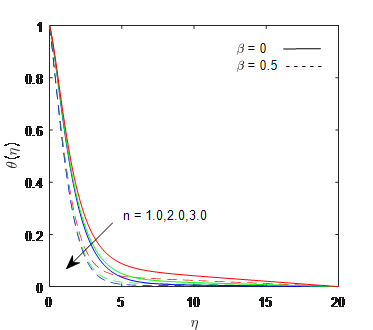Figure 12. θ(η) versus η for changed points of n when Le=1, M=0.2, Nt=0.2, Pr=1, γ=0.5, Nb=0.2 & Ec=0.5

## 13.png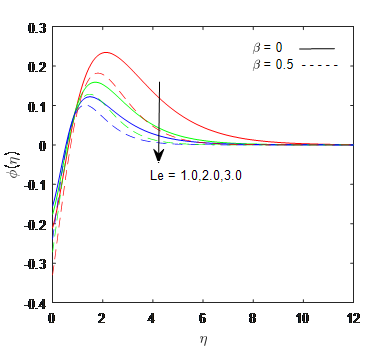Figure 13. ϕ(η) versus η for changed points of Le when n=0.5, M=0.2, Nt=0.2, Pr=1, γ=0.5, Nb=0.2 & Ec=0.5

5. Conclusions

In this paper explores the Casson MHD Nano fluid flow with internal heat generation and viscous dissipation of an exponential stretching sheet. In previous literature the effects of MHD Casson Nanofluid flow with internal heat generation and viscous dissipation of exponential stretching sheet is not appeared by author’s knowledge so for. The primary finishing up emphases are given below:

(1) The velocity depicts and energy transfer rate increase with enhance in magnetic field.

(2) The heat transfer rate increases with increase in Prandtl number Pr.

(3) By uplifting of thermophorosis Nt, the energy transfer rate enhances.

(4) The concentration decreases with increase in Lewis number.

Acknowledgment

Authors are thankful to the referee for the valuable suggestions who help to improve the quality of this manuscript and also support of our institutional departments.

References

 Okonkwo, E.C., Wole-Osho, I., Almanassra, I.W., Abdullatif, Y.M., Al-Ansari, T. (2021). An updated review of nanofluids in various heat transfer devices. Journal of Thermal Analysis and Calorimetry, 145(6): 2817-2872. https://doi.org/10.1007/s10973-020-09760-2

 Ganji, D.D., Sabzehmeidani, Y., Sedighiamiri, A. (2018). Nonlinear systems in heat transfer. Elsevier, 1: 12-28.

 Okonkwo, E.C., Wole-Osho, I., Kavaz, D., Abid, M. (2019). Comparison of experimental and theoretical methods of obtaining the thermal properties of alumina/iron mono and hybrid nanofluids. Journal of Molecular Liquids, 292: 111377. https://doi.org/10.1016/j.molliq.2019.111377

 Abbas, W., Magdy, M.M. (2020). Heat and mass transfer analysis of nanofluid flow based on, and over a moving rotating plate and impact of various nanoparticle shapes. Mathematical Problems in Engineering, 2020: 9606382. https://doi.org/10.1155/2020/9606382

 Zaidi, H.N., Yousif, M., Nazia Nasreen, S. (2020). Effects of thermal radiation, heat generation, and induced magnetic field on hydromagnetic free convection flow of couple stress fluid in an isoflux-isothermal vertical channel. Journal of Applied Mathematics, 2020: 4539531. https://doi.org/10.1155/2020/4539531

 Khan, W.A., Sun, H., Shahzad, M., Ali, M., Sultan, F., Irfan, M. (2021). Importance of heat generation in chemically reactive flow subjected to convectively heated surface. Indian Journal of Physics, 95(1): 89-97. https://doi.org/10.1007/s12648-019-01678-2

 Kemparaju, M.C., Lavanya, B., Nandeppanavar, M.M., Raveendra, N. (2021). Melting MHD stagnation point flow and heat transfer of a nano fluid with non-linear thermal radiation and chemical reaction. Psychology and Education, 58(2): 6489-6496.

 Lavanya, B. (2020). Hall current and thermal radiation effects on heat and mass transfer of unsteady MHD flow of a viscoelastic micropolar fluid through a porous medium. Journal of Advanced Research in Fluid Mechanics and Thermal Sciences, 68(1): 1-10.

 Noghrehabadi, A., Ghalambaz, M., Ghanbarzadeh, A. (2012). Heat transfer of magnetohydrodynamic viscous nanofluids over an isothermal stretching sheet. Journal of Thermophysics and Heat Transfer, 26(4): 686-689. https://doi.org/10.2514/1.T3866

 Mondal, S., Oyelakin, I.S., Sibanda, P. (2017). Unsteady MHD three-dimensional Casson nanofluid flow over a porous linear stretching sheet with slip condition. Frontiers in Heat and Mass Transfer (FHMT), 8: 37.

 Acharya, N., Maity, S., Kundu, P.K. (2020). Influence of inclined magnetic field on the flow of condensed nanomaterial over a slippery surface: the hybrid visualization. Applied Nanoscience, 10(2): 633-647. https://doi.org/10.1007/s13204-019-01123-0

 Sarifuddin. (2022). CFD modelling of Casson fluid flow and mass transport through atherosclerotic vessels. Differ Equ Dyn Syst, 30: 253-269. https://doi.org/10.1007/s12591-020-00522-y

 Sohail, M., Shah, Z., Tassaddiq, A., Kumam, P., Roy, P. (2020). Entropy generation in MHD Casson fluid flow with variable heat conductance and thermal conductivity over non-linear bi-directional stretching surface. Scientific Reports, 10(1): 1-16.

 Debnath, S., Saha, A.K., Mazumder, B.S., Roy, A.K. (2020). On transport of reactive solute in a pulsatile Casson fluid flow through an annulus. International Journal of Computer Mathematics, 97(11): 2303-2319. https://doi.org/10.1080/00207160.2019.1695047

 Raju, M.C., KVS, R. (2020). MHD Casson fluid flow through a vertical plate. Journal of Computational & Applied Research in Mechanical Engineering (JCARME), 9(2): 343-350. https://jcarme.sru.ac.ir/article_1101.html

 Swapna, M.N., Anusha, P., Chittaranjandas, V., Srividya, K. (2021). Radiation effect on MHD Casson fluid flow linearly porous stretching sheet in the presence chemical reaction. Annals of the Romanian Society for Cell Biology, pp. 4430-4439.

 Gharami, P.P., Arifuzzaman, S.M., Reza-E-Rabbi, S., Shakhaoath Khan, M., Ahmmed, S.F. (2020). Analytical and numerical solution of viscous fluid flow with the effects of thermal radiation and chemical reaction past a vertical porous surface. International Journal of Heat and Technology, 38(3): 689-700. https://doi.org/10.18280/ijht.380313